## Slugsnack’s Reversing Series 

Attached to this thread is the target we are reversing today (g4.exe) and also a keygen with source for it made by me.

Okie dokes. As always we open up our target in Olly and run it: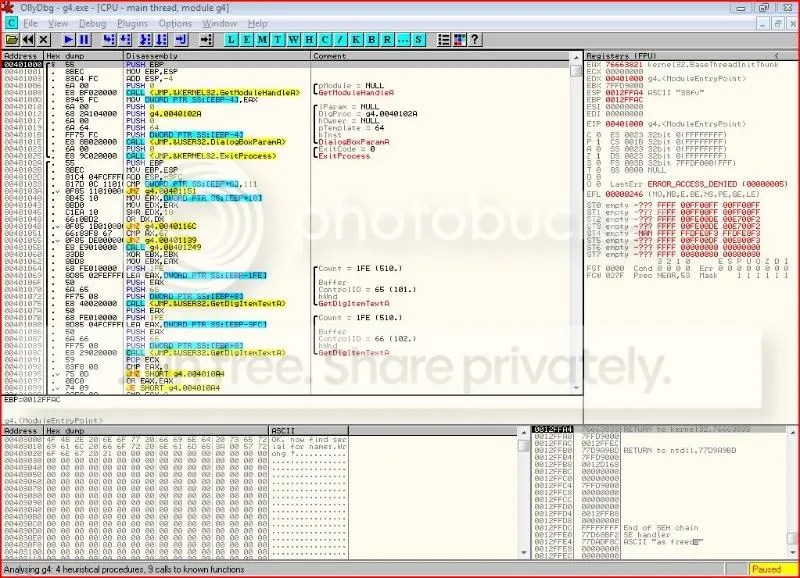Let’s try and put some garbage in and see what happens:So I will now start tracing the code from the point where my entries into the two dialogues are fetched. So we set a breakpoint on calls to GetDlgItemTextA: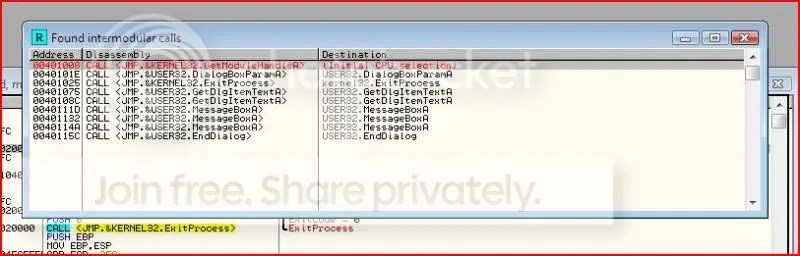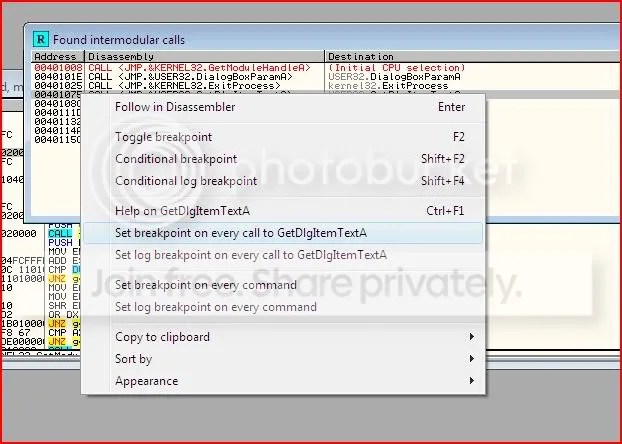The two different calls to GetDlgItemTextA represent the application copying the contents into a buffer for the username and the serial.

Again we enter our garbage and we break in Olly: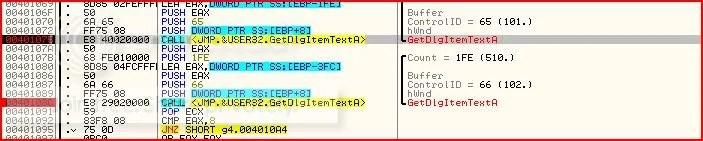We’ve looked at GetDlgItemTextA quite a few times now already so if you look a little further down: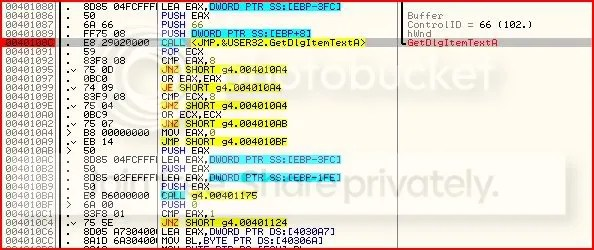You should be able to see that the length of the username and serial are required to be 8 characters long otherwise code execution jumps to the bad message.

Now, I don’t have an incredible memory so I find it helps a lot (especially on larger applications) to comment what’s happening as I’m going along so that I don’t forget. To label, double-click on the instruction’s line in the comment column and Olly will let you add a comment:To meet those requirements I will now change the username so it’s of the right number of characters:

Slugsnack >> Slugsnac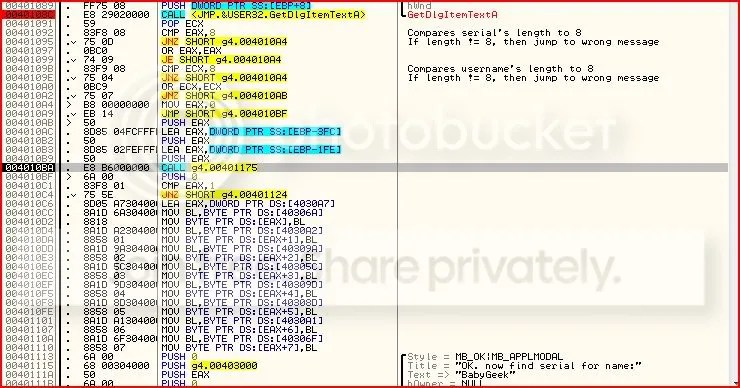I have stopped stepping the code at 004010BA. Why ? Well if you look 3 lines past that at 004010C4, you can see the following instruction:

`JNZ SHORT g4.00401124`

On closer inspection, this leads to the wrong message box: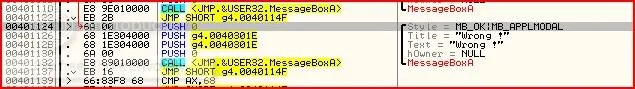So how could we avoid jumping here ? Well the result of that conditional jump is decided by the instruction at 004010C1:

`CMP EAX,1`

If EAX = 1, then the result of the compare would be that the zero flag would be set and therefore we would not jump. If EAX != 1, then the result of the compare would be that the zero flag is not set and we would jump to the bad message. Hence we want EAX to hold the value 1 prior to the compare.

Looking back at the call we stopped at, it should be obvious this is the last place EAX could be changed before the compare.

Stepping into the call: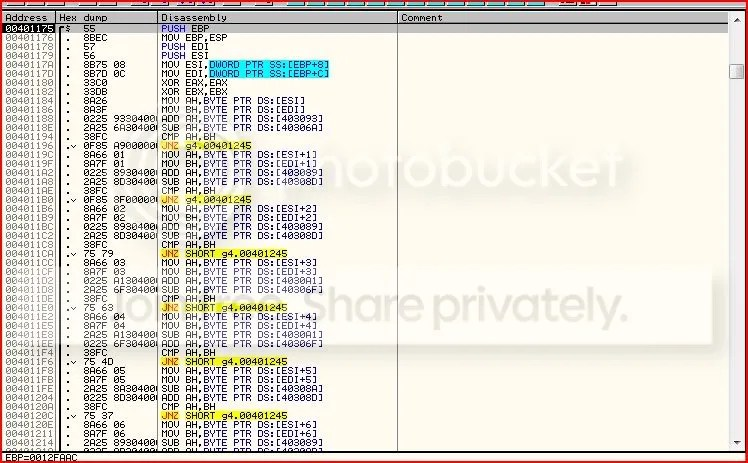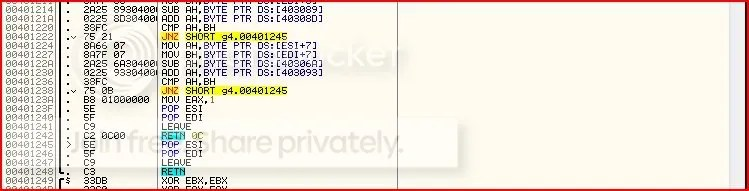Damn. That’s a lot.. However, looking closer, we can see that a bunch of instructions are repeated again and again. They seem to be in the form:

```MOV AH,*
MOV BH,*
SUB AH,*
CMP AH,BH
JNZ g4.00401245```

So AH is going through some sort of manipulation and then it’s being compared to BH. If they are not equal, execution jumps to 00401245: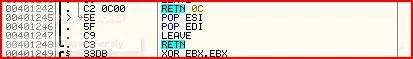If we jump here, we will result with EAX as 0 and eventually jump to the wrong message box after we return from the call because EAX is xor’red with itself further up at the start of the call.

Now let’s go back to the start of the call and see what exactly AH and BH are: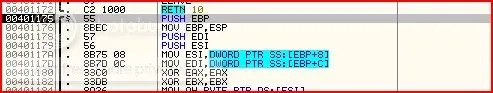I’ll leave you to look through the hex to see what EBP+8 and EBP+C hold but I’ll tell you generally what’s happening:
– EBP, EDI and ESI are pushed onto the stack which means that they’re likely to be used for calculations later (remember what I said in the last part of this series about how you need to make sure every register holds its initial values after you use them ?)
– Username (Slugsnac) is moved to ESI
– Serial (Password) is moved to EDI
– EAX and EBX are set to zero

Now let’s have a look at the first set of instructions: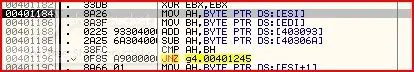So the first byte of ESI (first character of username) is moved to AH and the first byte of EDI moved to BH (first character of username). AH is then added to whatever is stored in 403093 and 40306A subtracted from that. AH and BH are then compared. If they are not equal then we jump to the bad message box. Stepping into each instruction in turn, we can see that 403093 holds the value ‘k’ (6B) and 40306A holds ‘B’ (42). So we can say this is happening:

`AH + k - B`

This can be simplified to:

`AH + )`

Reason ?

Therefore:

k – B = 6B – 42
= 29

Looking up 29 in an ASCII table, we find it is equal to ‘)’. To look at an ASCII table, go to:
http://www.asciitable.com/

Okay so basically we see that that sort of function was repeated time and time again for every character of the username. However the operands differ in that the value added and subtracted everytime is different. I’ve gone and done all the hard work and summarised this:

```First byte of username moved to AH. First byte of password moved to BH.```

Add ‘k’ (6B) to AH then subtract ‘B’ (42) = +29

Compare AH and BH. If not equal, then jump to wrong message box.

Second byte of username moved to AH.
Second byte of password moved to BH.

Add ‘a’ (61) to AH then subtract ‘e’ (65) = -4

Compare AH and BH. If not equal, then jump to wrong message box.

Third byte of username moved to AH.
Third byte of password moved to BH.

Add ‘a’ (61) to AH then subtract ‘e'(65) = -4

Compare AH and BH. If not equal, then jump to wrong message box.

Fourth byte of username moved to AH.
Fourth byte of password moved to BH.

Add ‘y’ (79) to AH then subtract ‘G’ (47) = +32

Compare AH and BH. If not equal, then jump to wrong message box.

Fifth byte of username moved to AH.
Fifth byte of password moved to BH.

Subtract ‘y’ (79) from AH then add ‘G’ (47) = -32

Compare AH and BH. If not equal, then jump to wrong message box.

Sixth byte of username moved to AH.
Sixth byte of password moved to BH.

Subtract ‘b’ (62) from AH then add ‘e’ (65) = +3

Compare AH and BH. If not equal, then jump to wrong message box.

Seventh byte of username moved to AH.
Seventh byte of password moved to BH.

Subtract ‘a’ (61) from AH then add ‘e’ (65) = +4

Compare AH and BH. If not equal, then jump to wrong message box.

Last byte of username moved to AH.
Last byte of password moved to BH.

Subtract ‘B’ (42) from AH then add ‘k’ (6B) = +29

Compare AH and BH. If not equal, then jump to wrong message box.

So I pretended I had the serial correct by changing the Z-flag temporarily (explained in the previous part of this series) and went to where we should get to if all was well: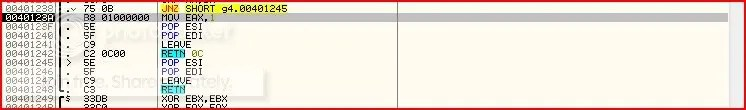EAX would be set as 1 and we’d return from the call and we would go to right message box !

So basically to cut a long story short, I programmed a keygen with high level assembly to do all the calculations/manipulations to me. I’ve included that as an attachment but I’ll lead you through it anyway. Use command prompt to navigate to the location of the keygen and type in keygen: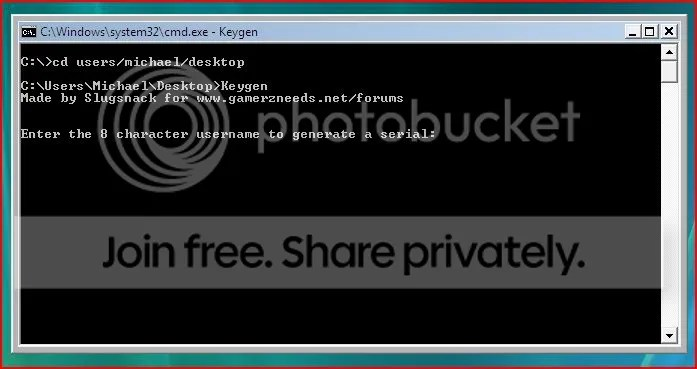Keep trying usernames until you get one where the output is in characters you can actually input:Run the application from the start and input the generated serial: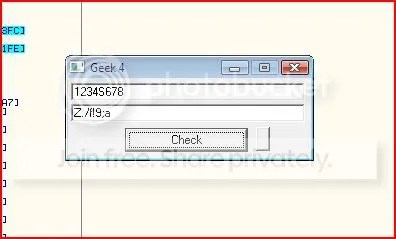Using my keygen again: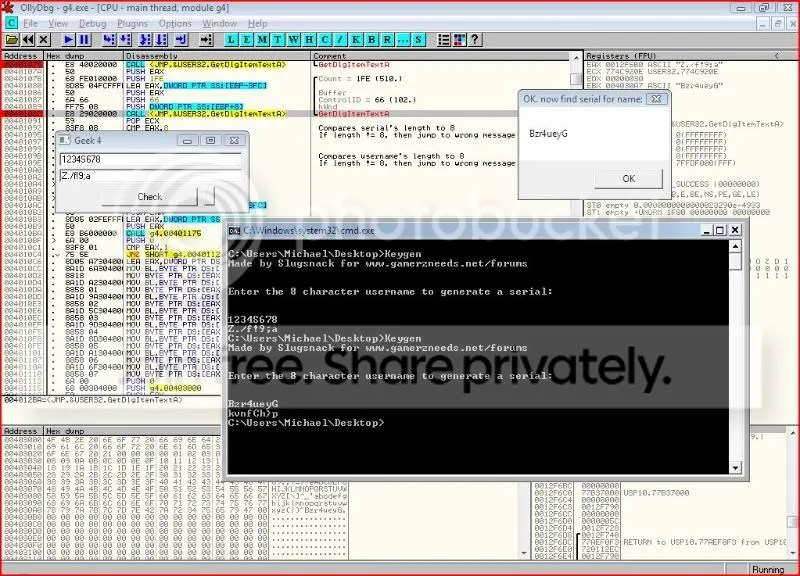Testing: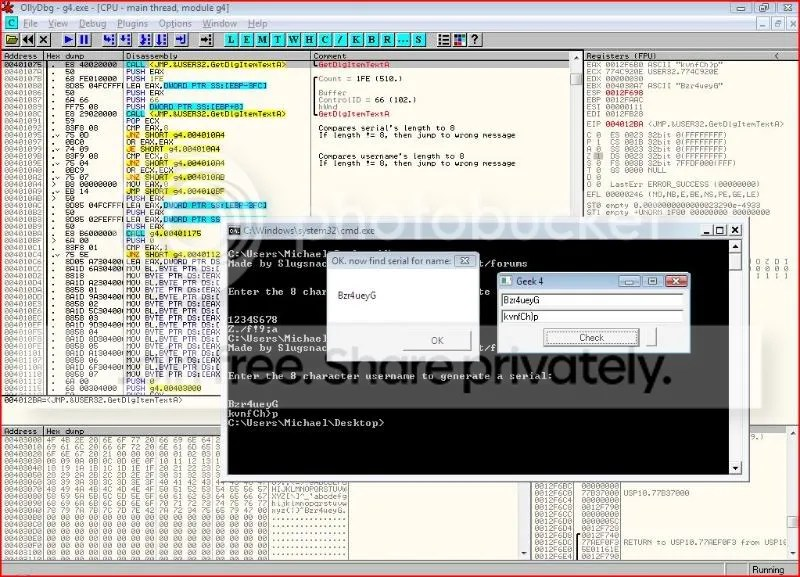Success ! And that’s the end of this reverseme. If you have little or no experience of programming this part of the series may have seemed dull since all you were doing was inputting something into a pre-made keygen but hopefully you will appreciate what is happening so you can do it for yourself next time 🙂